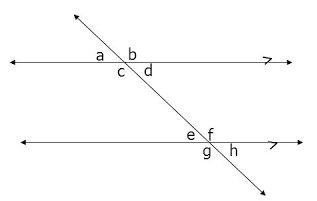# Third Term Examination Mathematics JSS 1 (Basic 7) – Exam Questions

MATHEMATICS

THIRD TERM EXAMINATION

JUNIOR SECONDARY SCHOOLS

JSS 1 (BASIC 7)

### SECTION A

1. Simplify 2a + 3A – 4a + 5a.

(a) 12a

(b) 14a

(c) 7a

(d) 6a

2. The place value of 3 in 43641 is __________.

(a) thousands

(b) hundreds

(c) tens

(d) unit

3. Ayo saves ₦50 daily, how much will he save for 10 days?

(a) ₦250

(b) ₦500

(c) ₦5,000

(d) ₦2,500

4. 13ten is equal to PTWO.

(a) 10010

(b) 1101

(c) 11010

(d) 1111

5. MMDCCIX is __________.

(a) 2709

(b) 2711

(c) 2309

(d) 2311

6. Express 0.0928 in 2 significant figures.

(a) 0.093

(b) 0.09

(c) 0.092

(d) 0.10

7. Simplify 3m + 4n – 5p – 2n – 3m

(a) 2n – 5p

(b) 6m + 2n – 5p

(c) 6m + 6n – 5p

(d) 1 – 2n + 5p

8. Solve for x in x + 2 = 12

(a) x = 8

(b) x = 10

(c) x = 12

(d) x = 6

9. __________ is a polygon with 3 sides and angles.

(a) Square

(b) Trapezium

(c) Triangle

(d) Rectangle

10. All are quadrilaterals except __________.

(a) square

(b) rhombus

(c) kite

(d) scalene

11. E means __________ in EFV.

(a) edges

(b) event

(c) effort

(d) ephraim

12. Solve for a in 15 + 2a = 23

(a) a = 8

(b) a = 4

(c) a = 16

(d) a = 2

13. __________ is the use of pictures to represent data given.

(a) Pictogram

(b) Pie chart

(c) Bar chart

(d) Histogram

14. Angle a and b are __________ angles.(a) complementary

(b) supplementary

(c) parallel

(d) alternate

15. __________ is the perimeter for square.

(a) l + b

(b) l + b + l +b

(c) l + l + l + l

(d) l x b

16. All are types of triangle except __________.

(a) isosceles

(b) right – angled

(c) scalene

(d) acute

17. The figure shown is a __________.

(a) cube

(b) cuboid

(c) cylinder

(d) cone

18. __________ is an example of 3-dimensional shape.

(a) Triangle

(b) Sphere

(c) Circle

(d) Rhombus

19. Change 7¼ to mixed fraction.

(a) 29/3

(b) 28/3

(c) 12/3

(d) 11/3

20. Add up 3/5 and 4/3 together.

(a) 28/15

(b) 7/8

(c) 114/15

(d) 2

### SECTION B

QUESTION 1

Draw any 3 three dimensional shapes and label.

QUESTION 2

Solve x in the equation:

A. 12+x= 13

B. 3x + 2a =13

QUESTION 4

A. What is polygon?

B. List any four types of triangle.

QUESTION 5

Translate to word problem:

A. 2+a=30

B. 3a+15=30

1.2.